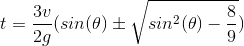# How Can I Get This Equation From These 3 Equations

Hi, I've Read an Article That Says:
If:
EQ.1:and EQ2:
http://latex.codecogs.com/gif.latex?x=vtcos(\theta)

and EQ3:
http://latex.codecogs.com/gif.latex?y=-\frac{1}{2}gt^2 &plus; vtsin(\theta)

We Can Get The Following Equation (an Ellipse Equation) By Substituting t(EQ1) into EQ.2 and EQ.3 Using StraightForward Algebra:
http://latex.codecogs.com/gif.latex?(y-\frac{v^2}{4g})^2 &plus; \frac{1}{4}x^2=(\frac{v^2}{4g})^2

But How it is Possible?!

Last edited:
I don't believe that the coordinates ##x## and ##y## defined here will satisfy an elliptical equation. These are the equations for the position of an object propelled into a ballistic trajectory on Earth. If you solve eq. 2 for ##t## and then substitute into eq. 3, you will get the equation of a parabola:

$$y= - \frac{g}{2v^2 \cos^2\theta} \left( x - \frac{ v^2 \sin(2\theta)}{2 g } \right)^2 + \frac{ v^2 \sin^2\theta}{2 g }.$$

This is an approximation to the true elliptical gravitational orbit of the object. The reason we don't get an ellipse is because ##x## is a flat-Earth coordinate that does not follow the direction of the true curvature of the Earth's surface. In polar coordinates the elliptical nature of the orbit should emerge. I believe that this article should have a correct analysis of the situation.

Thank you. But my teacher said he had proved the equation. But he didn't prove it t for me